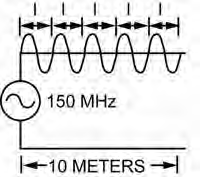# 3D153 Practice Exam

320 Questions | Total Attempts: 1807SettingsThis practice exam takes all URE questions from Vol 1 - 3 and randomly gives you 100 to answer. The questions are different every time you take this, so make sure to take this practice test more than once or twice! Hint: This is the MINIMUM you need to know. The number in parenthesis preceding each question gives you the section the answer can be found in. Be sure to read through each section!

• 1.
(201) Equipment used for generating, amplifying, and transmitting RF carrier is collectively called
• A.

• B.

An antenna.

• C.

A transmitter.

• D.

A transmission line.

• 2.
(201) What basic receiver function involves having the transmitted electromagnetic wave pass through the receive antenna in such a manner as to induce a voltage in the antenna?
• A.

Reproduction

• B.

Reception

• C.

Detection

• D.

Selection

• 3.
(201) The ability to reproduce the input signal accurately is characteristic of the receiver’s
• A.

Fidelity.

• B.

Capacity.

• C.

Sensitivity.

• D.

Selectivity.

• 4.
(202) A combination of a transmitter and receiver that is built as a single unit and sharing common tuned circuits is called a
• A.

Transmitter.

• B.

Transceiver.

• C.

• D.

Coupler.

• 5.
(202) Which of the following cannot be performed by the transceiver’s computerized components and micro-circuitry?
• A.

Specific equipment faults

• B.

Internal equipment tests

• C.

Internal calibrations

• D.

The ability to repair itself

• 6.
(203) A transmission line that consists of a center conductor placed inside a metal tube functioning as the outer shield is called a
• A.

Flexible coaxial cable.

• B.

Rigid coaxial cable.

• C.

Waveguide.

• D.

• 7.
(203) Which selection best describes the term “cutoff frequency” when discussing transmission line properties?
• A.

The frequency where XL becomes so low that the signal is shunted.

• B.

The frequency where XC causes the signal to be shunted.

• C.

The lowest frequency that the line can pass successfully.

• D.

The frequency at which standing waves are maximum.

• 8.
(203) Using figure 1–10, determine the wavelength if the frequency is changed from 150 MHz to 250 MHz.
• A.

12.0 meters.

• B.

3.0 meters.

• C.

1.5 meters.

• D.

1.2 meters.

• 9.
(203) Using figure 1–10, determine the electrical length if the frequency is changed from 150 MHz to 250 MHz.
• A.

8.33 meters.

• B.

8.33 wavelengths.

• C.

83.3 meters.

• D.

83.3 wavelengths.

• 10.
(203) A non-resonant transmission line is a line
• A.

Having reflected waves.

• B.

Having no reflected waves.

• C.

With maximum voltage across its open termination.

• D.

With maximum voltage across its shorted termination.

• 11.
(203) If a transmission line is terminated in a short,
• A.

Current is at maximum and voltage at minimum at the termination.

• B.

Current is at minimum and voltage at maximum at the termination.

• C.

There would be a nominal amount of signal gain.

• D.

The line would be non-resonant.

• 12.
(204) In a basic communication system, what converts radio frequency (RF) energy’s current oscillation into electric and magnetic fields of force?
• A.

Antenna.

• B.

Coupler.

• C.

Transmitter.

• D.

Transmission lines.

• 13.
(204) The concept that alternating current (AC) changes in magnitude and reverses its direction during each cycle is
• A.

An unproven hypothesis.

• B.

The definition of propagation.

• C.

• D.

What led to the discovery of direct current.

• 14.
(205) If the electric field component travels in a plane perpendicular to the Earth’s surface, the radio wave is
• A.

Magnetically polarized.

• B.

Horizontally polarized.

• C.

Circularly polarized.

• D.

Vertically polarized.

• 15.
(205) What types of polarization do satellite terminals transmit and receive?
• A.

Transmit horizontal and receive vertical polarizations.

• B.

Transmit vertical and receive horizontal polarizations.

• C.

Transmit right hand and receive left hand circular polarizations.

• D.

Transmit left hand and receive right hand circular polarizations.

• 16.
(206) What measurement is used to determine whether an antenna is resonant at a particular frequency?
• A.

Distortion.

• B.

Standing wave ratio.

• C.

Signal-to-noise ratio.

• D.

Percent of modulation.

• 17.
(206) The ability of an antenna to both receive and transmit equally well is known as the antenna’s
• A.

Bandwidth.

• B.

Resonance.

• C.

Reciprocity.

• D.

Effectiveness.

• 18.
(206) The standard used to measure the radiating effectiveness (gain) of an antenna system is the
• A.

Marconi antenna.

• B.

Isotropic antenna.

• C.

Hertz antenna.

• D.

Whip antenna.

• 19.
(206) What does the effectiveness of an entire transmitting and receiving system depend largely upon?
• A.

Impedance matching.

• B.

Antenna distance.

• C.

Antenna properties.

• D.

Line characteristics.

• 20.
• A.

Omnidirectional.

• B.

Unidirectional.

• C.

Bidirectional.

• D.

Directional.

• 21.
(207) Which antenna type is usually used on long-range, point-to-point circuits where the concentrated radio energy is needed for circuitry reliability?
• A.

Omnidirectional.

• B.

Unidirectional.

• C.

Bidirectional.

• D.

Circular.

• 22.
(208) Ungrounded lengths of wire specifically designed to be either a half-wavelength or more than full wavelength long is called a
• A.

Reflector.

• B.

Hertz antenna.

• C.

Marconi antenna.

• D.

Vertical monopole.

• 23.
(209) A long-wire’s takeoff angle depends on the antenna’s
• A.

Length.

• B.

Directivity.

• C.

Front-to-back ratio.

• D.

Standing wave ratio.

• 24.
(209) All antennas used in satellite communications are designed to be
• A.

Circular directional.

• B.

Omnidirectional.

• C.

Bidirectional.

• D.

Directional.

• 25.
(209) A common satellite antenna is the
• A.

Whip.

• B.

Discone.

• C.

Parabolic.

• D.

Long-wire.

Related Topics